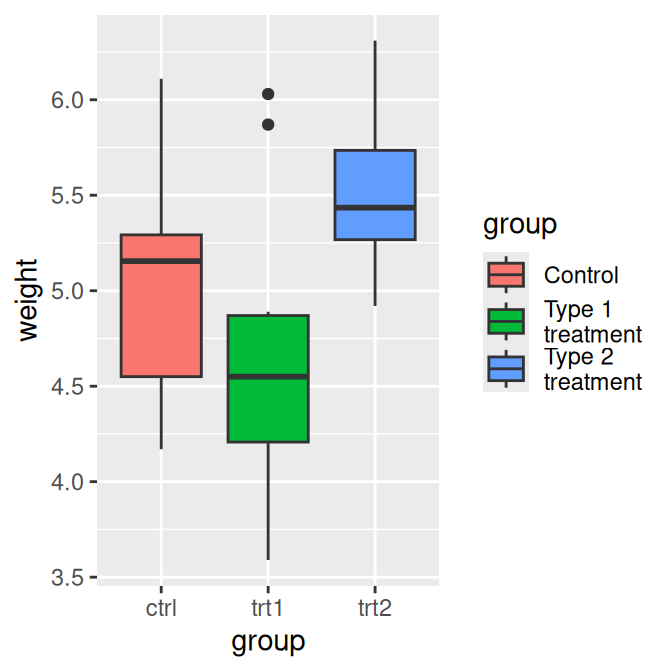## 10.10 Using Labels with Multiple Lines of Text

### 10.10.1 Problem

You want to use legend labels that have more than one line of text.

### 10.10.2 Solution

Set the labels in the scale, using `\n` to represent a newline. In this example, we’ll use `scale_fill_discrete()` to control the legend for the fill scale (Figure 10.17, left):

``````pg_plot <- ggplot(PlantGrowth, aes(x = group, y = weight, fill = group)) +
geom_boxplot()

# Labels that have more than one line
pg_plot +
scale_fill_discrete(labels = c("Control", "Type 1\ntreatment", "Type 2\ntreatment"))``````

### 10.10.3 Discussion

As you can see in the version on the left in Figure 10.17, with the default settings the lines of text will run into each other when you use labels that have more than one line. To deal with this problem, you can increase the height of the legend keys and decrease the spacing between lines, using `theme()` (Figure 10.17, right). To do this, you will need to specify the height using the `unit()` function from the grid package:

``````library(grid)

pg_plot +
scale_fill_discrete(labels = c("Control", "Type 1\ntreatment", "Type 2\ntreatment")) +
theme(legend.text = element_text(lineheight = .8), legend.key.height = unit(1, "cm"))``````Figure 10.17: Multiline legend labels (left); With increased key height and reduced line spacing (right)